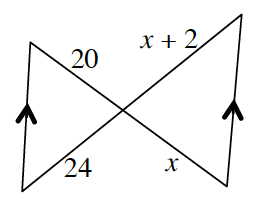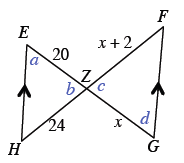### Home > CCG > Chapter 6 > Lesson 6.2.3 > Problem6-73

6-73.Examine the triangles in the diagram at right.

1. Are the triangles similar? If you decide that they are, then justify your conclusion using a flowchart.

There are some parallel lines in the diagram.
Review Math Notes in Lesson 2.1.4 for help identifying the relationships between the angles lettered $a$, $b$, $c$, and $d$ below.2. Solve for $x$. Show all work.

When two triangles are similar, the ratios of corresponding sides are equal.
You could use a proportion.

$x=10$